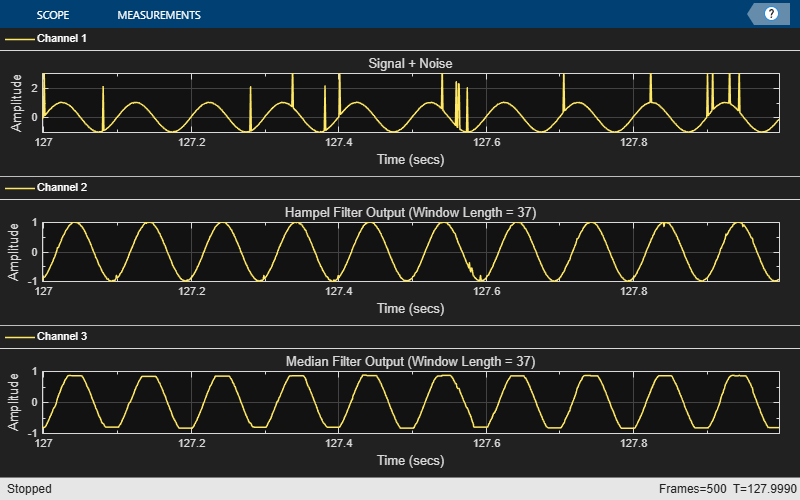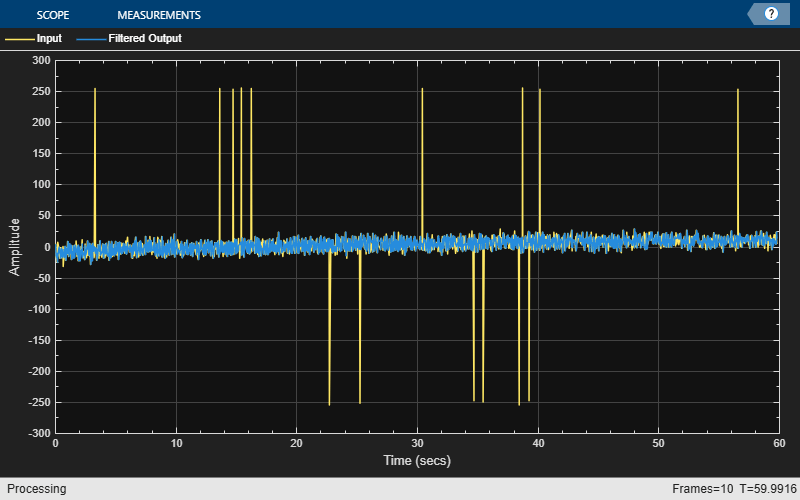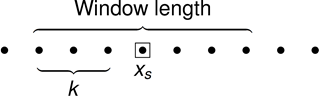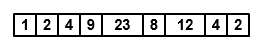# dsp.HampelFilter

Filter outliers using Hampel identifier

## Description

The `dsp.HampelFilter` System object™ detects and removes the outliers of the input signal by using the Hampel identifier. The Hampel identifier is a variation of the three-sigma rule of statistics that is robust against outliers. For each sample of the input signal, the object computes the median of a window composed of the current sample and $\frac{Len-1}{2}$ adjacent samples on each side of current sample. Len is the window length you specify through the `WindowLength` property. The object also estimates the standard deviation of each sample about its window median by using the median absolute deviation. If a sample differs from the median by more than the threshold multiplied by the standard deviation, the filter replaces the sample with the median. For more information, see Algorithms.

To filter the input signal using a Hampel identifier:

1. Create the `dsp.HampelFilter` object and set its properties.

2. Call the object with arguments, as if it were a function.

## Creation

### Syntax

``hampFilt = dsp.HampelFilter``
``hampFilt = dsp.HampelFilter(Len)``
``hampFilt = dsp.HampelFilter(Len, Lim)``
``hampFilt = dsp.HampelFilter(Name,Value)``

### Description

````hampFilt = dsp.HampelFilter` returns a Hampel filter object, `hampFilt`, using the default properties.```

example

````hampFilt = dsp.HampelFilter(Len)` sets the `WindowLength` property to `Len`.```
````hampFilt = dsp.HampelFilter(Len, Lim)` sets the `WindowLength` property to `Len` and the `Threshold` property to `Lim`.Example: `hampFilt = dsp.HampelFilter(11,2);````
````hampFilt = dsp.HampelFilter(Name,Value)` specifies properties using `Name,Value` pairs. Unspecified properties have default values.```

## Properties

expand all

Unless otherwise indicated, properties are nontunable, which means you cannot change their values after calling the object. Objects lock when you call them, and the `release` function unlocks them.

If a property is tunable, you can change its value at any time.

If a property is listed as tunable, then you can change its value even when the object is locked.

Length of the sliding window, specified as a positive odd scalar integer. The window of finite length slides over the data, and the object computes the median and median absolute deviation of the data in the window.

Data Types: `single` | `double`

Threshold for outlier detection, specified as a positive real scalar. For information on how this property is used to detect the outlier, see Algorithms.

Tunable: Yes

Data Types: `single` | `double`

## Usage

### Syntax

``y = hampFilt(x)``
``[y,isOutlier] = hampFilt(x)``

### Description

example

````y = hampFilt(x)` detects and removes the outliers of each channel of the input signal, `x`, independently over time using the Hampel filter.```
````[y,isOutlier] = hampFilt(x)` returns a logical array, `isOutlier`, in which each `true` element indicates that the corresponding element in the input is an outlier. `isOutlier` is the same size as the input and output vectors.```

### Input Arguments

expand all

Data input, specified as a vector or a matrix. The object accepts multichannel inputs, that is, m-by-n size inputs, where m ≥ 1, and n > 1. m is the number of samples in each frame (channel), and n is the number of channels. The object also accepts variable-size inputs. After the object is locked, you can change the size of each input channel, but you cannot change the number of channels.

Data Types: `single` | `double`

### Output Arguments

expand all

Filtered data, returned as a vector or a matrix.

Data Types: `single` | `double`

Logical array whose elements indicate if the corresponding element in the input array is an outlier. If an element in `isOutlier` is `true`, the corresponding element in the input array is an outlier.

Data Types: `logical`

## Object Functions

To use an object function, specify the System object as the first input argument. For example, to release system resources of a System object named `obj`, use this syntax:

`release(obj)`

expand all

 `step` Run System object algorithm `release` Release resources and allow changes to System object property values and input characteristics `reset` Reset internal states of System object

## Examples

collapse all

Filter high-frequency noise from a noisy sine wave signal using a Hampel filter. Compare the performance of the Hampel filter with a median filter.

Initialization

Set up a `dsp.HampelFilter` and a `dsp.MedianFilter` object. These objects use the sliding window method with a window length of 7. Create a time scope for viewing the output.

```Fs = 1000; hampFilt = dsp.HampelFilter(7); medFilt = dsp.MedianFilter(7); scope = timescope('SampleRate',Fs, ... 'TimeSpanOverrunAction','Scroll', ... 'TimeSpanSource','Property',... 'TimeSpan',1,'ShowGrid',true, ... 'LayoutDimensions',[3 1], ... 'NumInputPorts',3); scope.ActiveDisplay = 1; scope.Title = 'Signal + Noise'; scope.YLimits = [-1 3]; scope.ActiveDisplay = 2; scope.Title = 'Hampel Filter Output (Window Length = 7)'; scope.YLimits = [-1 1]; scope.ActiveDisplay = 3; scope.Title = 'Median Filter Output (Window Length = 7)'; scope.YLimits = [-1 1]; ```

Filter the Noisy Sine Wave Using a Window of Length 7

Generate a noisy sine wave signal with a frequency of 10 Hz. Apply the Hampel filter and the median filter object to the signal. View the output on the time scope.

```FrameLength = 256; sine = dsp.SineWave('SampleRate',Fs,'Frequency',10,... 'SamplesPerFrame',FrameLength); for i = 1:500 hfn = 3 * (rand(FrameLength,1) < 0.02); x = sine() + 1e-2 * randn(FrameLength,1) + hfn; y1 = hampFilt(x); y2 = medFilt(x); scope(x,y1,y2); end release(scope) ```Both filters remove the high-frequency noise. However, when you increase the window length, the Hampel filter is preferred. Unlike the median filter, the Hampel filter preserves the shape of the sine wave even with large window lengths.

Filter the Noisy Sine Wave Using a Window of Length 37

Increase the window length of both the filters to 37. Filter the noisy sine wave and view the filtered output on the time scope. To change the window length of the filters, you must release the filter objects at the start of the processing loop.

```release(hampFilt); release(medFilt); hampFilt.WindowLength = 37; medFilt.WindowLength = 37; scope.ActiveDisplay = 1; scope.Title = 'Signal + Noise'; scope.ActiveDisplay = 2; scope.Title = 'Hampel Filter Output (Window Length = 37)'; scope.ActiveDisplay = 3; scope.Title = 'Median Filter Output (Window Length = 37)'; for i = 1:500 hfn = 3 * (rand(FrameLength,1) < 0.02); x = sine() + 1e-2 * randn(FrameLength,1) + hfn; y1 = hampFilt(x); y2 = medFilt(x); scope(x,y1,y2); end release(scope) ```The median filter flattens the crests and troughs of the sine wave due to the median operation over a large window of data. The Hampel filter preserves the shape of the signal, in addition to removing the outliers.

Remove the high-frequency outliers from a streaming signal using the `dsp.HampelFilter` System object™.

Use the `dsp.MatFileReader` System object to read the gyroscope MAT file. The file contains three columns of data, with each column containing 7140 samples. The three columns represent the x-axis, y-axis, and z-axis data from the gyroscope motion sensor. Choose a frame size of 714 samples so that each column of the data contains 10 frames. The `dsp.HampelFilter` System object uses a window length of 11. Create a `timescope` object to view the filtered output.

```reader = dsp.MatFileReader('SamplesPerFrame',714,'Filename','LSM9DSHampelgyroData73.mat', ... 'VariableName','data'); hampFilt = dsp.HampelFilter(11); scope = timescope('NumInputPorts',1,'SampleRate',119,'YLimits',[-300 300], ... 'ChannelNames',{'Input','Filtered Output'},... 'TimeSpanSource','Property','TimeSpan',60,'ShowLegend',true); ```

Filter the gyroscope data using the `dsp.HampelFilter` System object. View the filtered z-axis data in the Time Scope.

```for i = 1:10 gyroData = reader(); filteredData = hampFilt(gyroData); scope([gyroData(:,3),filteredData(:,3)]); end ```The Hampel filter removes all the outliers and preserves the shape of the signal.

expand all

## AlgorithmsFor a given sample of data, xs, the algorithm:

• Centers the window of odd length at the current sample.

• Computes the local median, mi, and standard deviation, σi, over the current window of data.

• Compares the current sample with nσ × σi, where nσ is the threshold value. If $|{x}_{s}-{m}_{i}|>{n}_{\sigma }×{\sigma }_{i}$, the filter identifies the current sample, xs, as an outlier and replaces it with the median value, mi.

Consider a frame of data that is passed into the Hampel filter.In this example, the Hampel filter slides a window of length 5 (Len) over the data. The filter has a threshold value of 2 (nσ). To have a complete window at the beginning of the frame, the filter algorithm prepends the frame with Len – 1 zeros. To compute the first sample of the output, the window centers on the ${\left[\frac{Len-1}{2}+1\right]}^{\text{th}}$ sample in the appended frame, the third zero in this case. The filter computes the median, median absolute deviation, and the standard deviation over the data in the local window.• Current sample: xs = 0.

• Window of data: win = [0 0 0 0 1].

• Local median: mi = median([0 0 0 0 1]) = 0.

• Median absolute deviation: $ma{d}_{i}=\mathrm{median}\left(|{x}_{i-k}-{m}_{i}|,\dots ,|{x}_{i+k}-{m}_{i}|\right)$. For this window of data, $mad=\mathrm{median}\left(|0-0|,\dots ,|1-0|\right)=0$.

• Standard deviation: σi = κ × madi = 0, where $\kappa =\frac{1}{\sqrt{2}{\mathrm{erfc}}^{-1}\left(1/2\right)}\approx 1.4826$.

• The current sample, xs = 0, does not obey the relation for outlier detection.

`$\left[|{x}_{s}-{m}_{i}|=0\right]>\left[\left({n}_{\sigma }×{\sigma }_{i}\right)=0\right]$`

Therefore, the Hampel filter outputs the current input sample, xs = 0.

Repeat this procedure for every succeeding sample until the algorithm centers the window on the ${\left[End-\frac{Len-1}{2}\right]}^{\text{th}}$ sample, marked as `End`. Because the window centered on the last $\frac{Len-1}{2}$ samples cannot be full, these samples are processed with the next frame of input data.

Here is the first output frame the Hampel filter generates:The seventh sample of the appended input frame, 23, is an outlier. The Hampel filter replaces this sample with the median over the local window [4 9 23 8 12].

 Bodenham, Dean. “Adaptive Filtering and Change Detection for Streaming Data.” PH.D. Thesis. Imperial College, London, 2012.

 Liu, Hancong, Sirish Shah, and Wei Jiang. “On-line outlier detection and data cleaning.” Computers and Chemical Engineering. Vol. 28, March 2004, pp. 1635–1647.

## SupportGet trial now